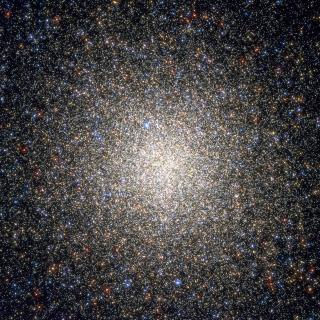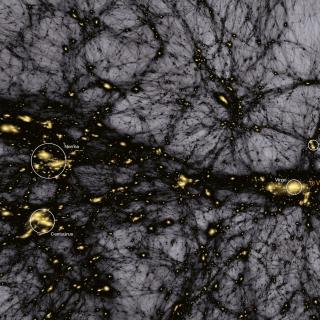# Exact Semianalytical Calculation of Rotation Curves with Bekenstein-Milgrom Nonrelativistic MOND

López-Corredoira, M.; Betancort-Rijo, J. E.
Referencia bibliográfica

The Astrophysical Journal

Fecha de publicación:
3
2021
Número de autores
2
Número de autores del IAC
2
Número de citas
4
Número de citas referidas
3
Descripción
Astronomers derive MOdified Newtonian Dynamics (MOND) rotation curves using the simple algebraic rule of calculating the acceleration as equal to the Newtonian acceleration (a) divided by some factor μ(a). However, there are velocity differences between this simple rule and the calculation derived from more sophisticated MOND versions such as AQUAL or QMOND, created to expand MOND heuristic law and preserve the conservation of momentum, angular momentum, and energy, and follow the weak equivalence principle. Here we provide recipes based on Milgrom's proposal to calculate semianalytically (without numerical simulations) MOND rotation curves for any density distribution based on AQUAL, applying it to different models of thin disks. The application of this formalism is equivalent to the creation of a fictitious phantom mass whose field may be used in a Newtonian way to calculate iteratively the MOND accelerations. In most cases, the differences between the application of the simple algebraic rule and the AQUAL-MOND calculations are small, ≲5%. However, the error of the algebraic solution is larger than 5% when more than half of the mass is in the MONDian regime (where Newtonian and MOND rotation speeds differ by more than 10%), reaching in some cases >70% discrepancy, such as in Maclaurin disks, representative of galaxies for which the rotational velocity rises to the edge of the disk as is seen in irregular galaxies. The slope of the rotation speed in the dependence with the radius or the vertical distance of the plane is also significantly changed.Vía Láctea y galaxias cercanas

El objetivo general del Proyecto es el estudio de la estructura, historia evolutiva y proceso de formación de galaxias a través de sus poblaciones estelares resueltas, tanto a partir de fotometría como espectroscopia. El proyecto puede dividirse en cuatro líneas principales: I. Historia de formación estelar en el Grupo Local. El objetivo de esta

Martín
López CorredoiraCosmología con Trazadores de la Estructura a Gran Escala del Universo

El Fondo Cósmico de Microondas (FCM) contiene la información estadística de las semillas primigenias que han dado lugar a la formación de todas las estructuras en el Universo. Su contrapartida natural en el Universo local es la distribución de las galaxias que surgen como resultado del crecimiento gravitatorio de aquellas fluctuaciones de densidad

FRANCISCO SHU
KITAURA JOYANES